The MODEL Procedure

Wald-based and likelihood-ratio-based confidence intervals are available in the MODEL procedure for computing a confidence interval on an estimated parameter. A confidence interval on a parameter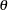can be constructed by inverting a Wald-based or a likelihood-ratio-based test.

The approximate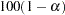% Wald confidence interval for a parameteris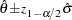where
is the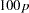th percentile of the standard normal distribution,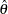is the maximum likelihood estimate of, and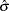is the standard error estimate of.

A likelihood-ratio-based confidence interval is derived from the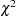distribution of the generalized likelihood ratio test. The approximate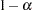confidence interval for a parameteris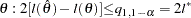where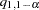is the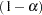quantile of thewith one degree of freedom, and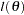is the log likelihood as a function of one parameter. The endpoints of a confidence interval are the zeros of the function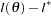. Computing a likelihood-ratio-based confidence interval is an iterative process. This process must be performed twice for each parameter, so the computational cost is considerable. Using a modified form of the algorithm recommended by Venzon and Moolgavkar (1988), you can determine that the cost of each endpoint computation is approximately the cost of estimating the original system.

To request confidence intervals on estimated parameters, specify the PRL= option in the FIT statement. By default, the PRL option produces 95% likelihood ratio confidence limits. The coverage of the confidence interval is controlled by the ALPHA= option in the FIT statement.

The following is an example of the use of the confidence interval options.

```   data exp;
do time = 1 to 20;
y = 35 * exp( 0.01 * time ) + 5*rannor( 123 );
output;
end;
run;

proc model data=exp;
parm zo 35 b;
dert.z = b * z;
y=z;
fit y init=(z=zo) / prl=both;
test zo = 40.475437 ,/ lr;
run;
```

The output from the requested confidence intervals and the TEST statement are shown in Figure 18.56

Figure 18.56 Confidence Interval Estimation
The MODEL Procedure

Nonlinear OLS Parameter Estimates
Parameter Estimate Approx Std Err t Value Approx
Pr > |t|
zo 36.58933 1.9471 18.79 <.0001
b 0.006497 0.00464 1.40 0.1780

Test Results
Test Type Statistic Pr > ChiSq Label
Test0 L.R. 3.81 0.0509 zo = 40.475437

Parameter Wald
95% Confidence Intervals
Parameter Value Lower Upper
zo 36.5893 32.7730 40.4056
b 0.00650 -0.00259 0.0156

Parameter Likelihood Ratio
95% Confidence Intervals
Parameter Value Lower Upper
zo 36.5893 32.8381 40.4921
b 0.00650 -0.00264 0.0157

Note that the likelihood ratio test reported the probability that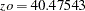is 5% butis the upper bound of a 95% confidence interval. To understand this conundrum, note that the TEST statement is using the likelihood ratio statistic to test the null hypothesis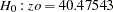with the alternate that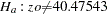. The upper confidence interval can be viewed as a test with the null hypothesis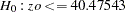.Previous Page | Next Page | Top of Page